# Kinematics Quiz 2

#### Quiz Description

In this second quiz, we shall go deeper into the study of kinematics. More emphasis will be laid on speed and velocity - time graphs and terminal velocity. Graphs are something very common you would find in this topic, implying that knowing it to your fingertips is a plus for you. Answering the quiz will give you an upper hand in the understanding of graph analysis and the fundamentals of kinematics.

Kinematics is a branch of mechanics that studies the motion of a point, body, or group of objects, without considering the cause of the motion. This topic greatly focuses on aspects like acceleration and velocity. To describe the motion of celestial bodies, astrophysicists make use of kinematics. Kinematics could also be applied in biomechanics by describing the motion of the skeletal system.

Now we have come to realize how important this quiz is to anyone studying kinematics. Are you looking for a way of understanding this topic? All you need to do is take this A level physics quiz and challenge yourself. Good luck!

A cyclist travels along a hilly road without using the pedals or brakes. Air resistance and friction are negligible. The speed / time graph of the cyclist is shown.

At which point did he reach the bottom of the first hill?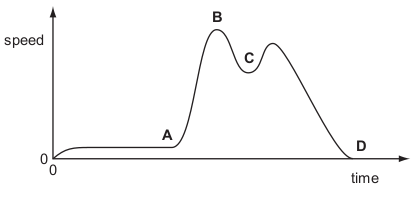Correct
• A
• B
• C
• D

A ball starts to roll down a steep slope and then along rough horizontal ground.

Which graph best shows the speed of the ball?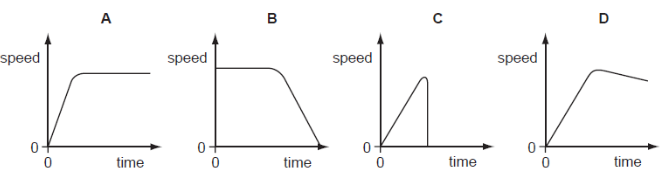Correct
• A
• B
• C
• D

A table-tennis ball is released from the top of an evacuated tube.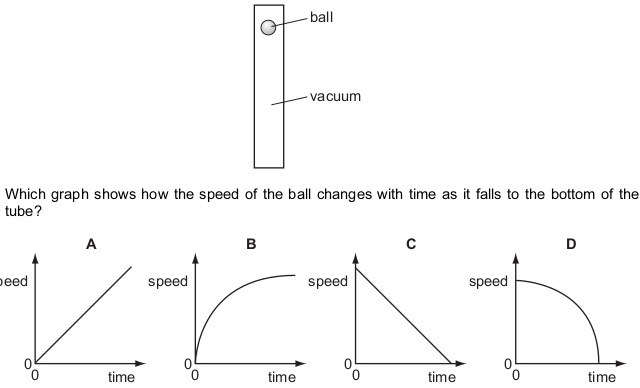Correct
• A
• B
• C
• D

A skydiver falls from rest through the air and reaches terminal velocity.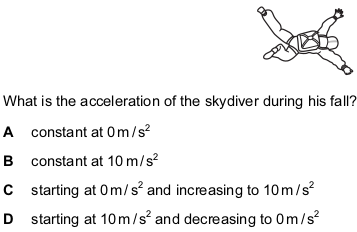Correct
• A
• B
• C
• D

A car accelerates from traffic lights for 10 s. It stays at a steady speed for 20 s and then brakes to a stop in 3 s.

Which graph shows the journey?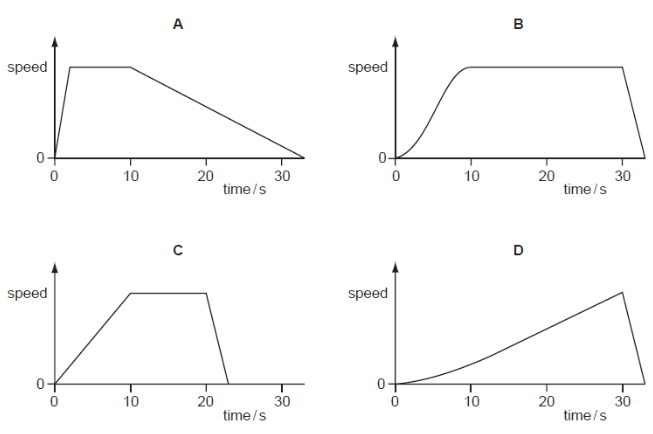Correct
• A
• B
• C
• D

Which vehicle has an acceleration of 5 m / s 2 ?

Correct
• a bicycle, when its speed changes from rest to 2.5 m / s in 2 s
• a car, when its speed changes from rest to 15 m / s in 5 s
• a lorry, when its speed changes from rest to 20 m / s in 15 s
• a motorbike, when its speed changes from rest to 50 m / s in 10 s

A car is stationary at a set of traffic lights.

When the lights turn green, the car begins to move and continues to speed up until it reaches the

maximum speed allowed. It continues to travel at this constant speed for the rest of the journey.

What happens to the acceleration and to the velocity of the car during this journey?

Correct
• Both the acceleration and the velocity change.
• Only the acceleration changes.
• Only the velocity changes.
• Neither the acceleration nor the velocity changes.

The speed-time graph for a car is shown.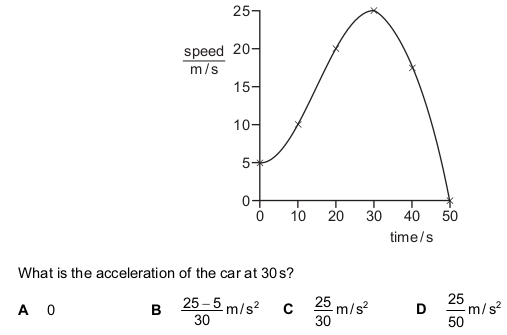Correct
• A
• B
• C
• D

The graph shows part of a journey made by a cyclist.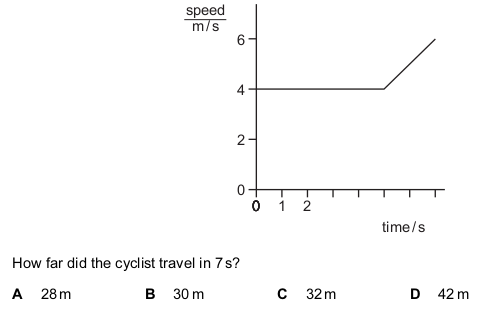Correct
• A
• B
• C
• D

The diagrams show a parachutist in four positions after she jumps from a high balloon.

At which position does she have terminal velocity?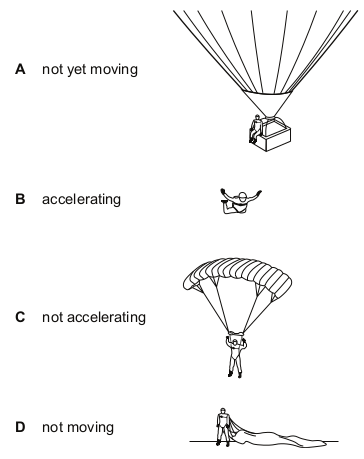Correct
• A
• B
• C
• D

Two men jump out of an aeroplane at the same time. One of the men opens his parachute and

the other man remains in free-fall. Why is the man in free-fall moving faster than the parachutist?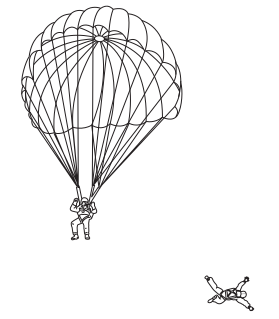Correct
• The man in free-fall experiences greater air resistance.
• The man in free-fall has a greater mass.
• The parachutist experiences greater air resistance.
• The parachutist has not reached terminal velocity.

A student measures the speed of a trolley. At one instant, the speed of the trolley is 1.0 m / s and two seconds later the speed is 4.0 m / s.

What is the acceleration of the trolley?

Correct
• 1.5 m / s 2
• 2.0 m / s 2
• 2.5 m / s 2
• 5.0 m / s 2

The speed-time graph shows the movement of a car. What does the shaded area of the graph represent?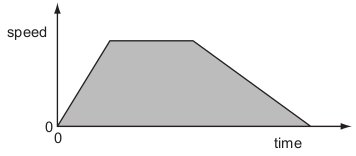Correct
• the average acceleration of the car
• the average speed of the car
• the total distance travelled by the car
• the total travelling time of the car

A small stone is dropped from the top of a ladder, falls and hits the ground. It does not rebound.

Which speed-time graph is correct?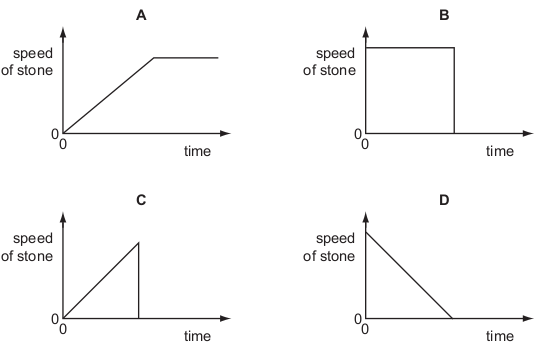Correct
• A
• B
• C
• D

A free-fall parachutist falls at a constant speed. He then opens his parachute and continues to fall to Earth at a lower, constant speed.

Which diagram shows how the distance fallen by the parachutist varies with time?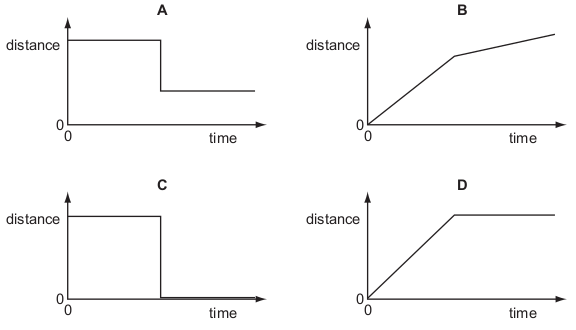Correct
• A
• B
• C
• D

The graph shows the speed of a runner during a race.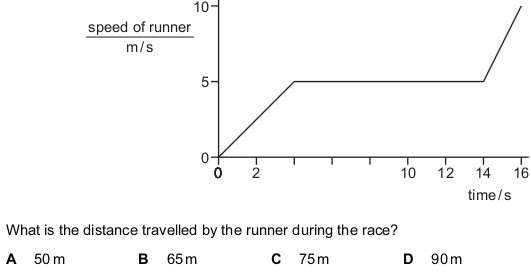Correct
• A
• B
• C
• D

Which speed-time graph shows an object moving with non-uniform acceleration?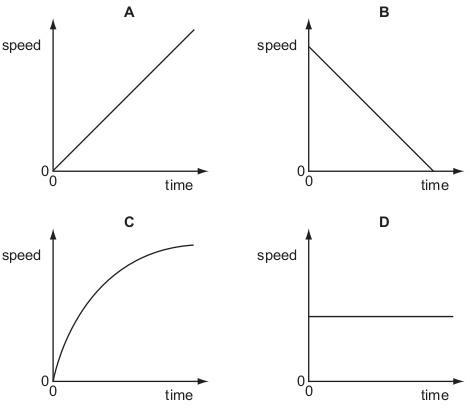Correct
• A
• B
• C
• D

The graph shows the speed of a car as it moves from rest.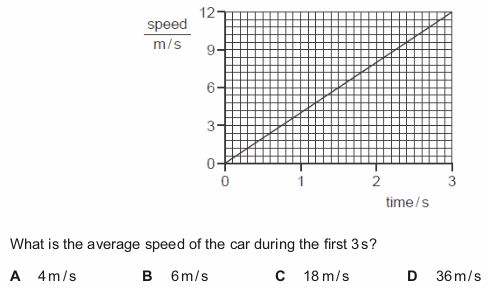Correct
• A
• B
• C
• D

A skier is traveling downhill. The acceleration on hard snow is 4 m / s 2 and on soft snow is 2 m / s 2 .

Which graph shows the motion of the skier when moving from hard snow to soft snow?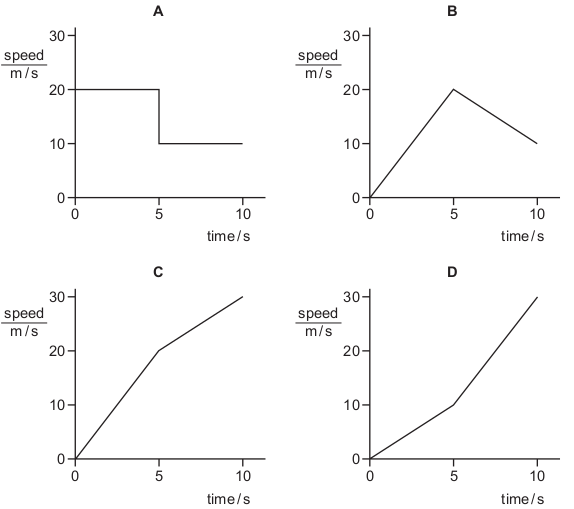Correct
• A
• B
• C
• D

The diagram represents the Moon in its orbit around the Earth.

Which arrow represents the direction of the resultant force acting on the Moon at the instant

shown?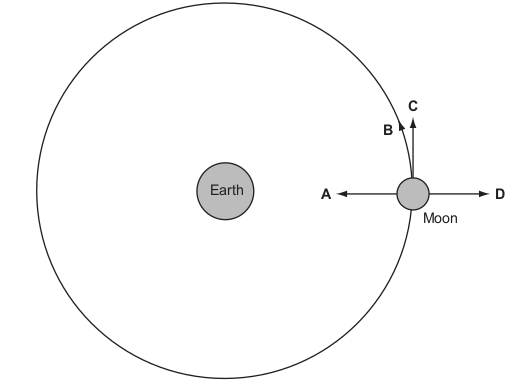Correct
• A
• B
• C
• D